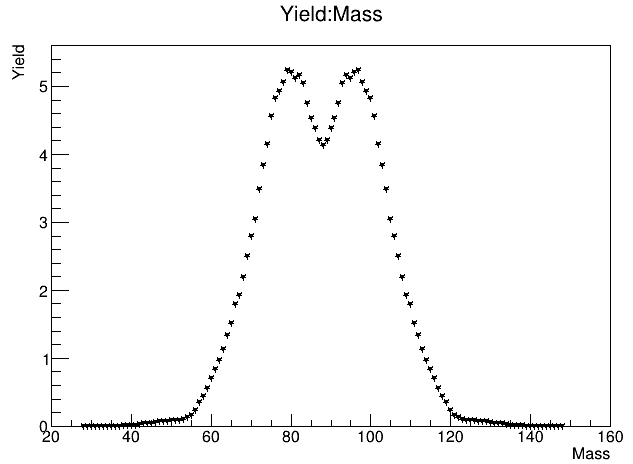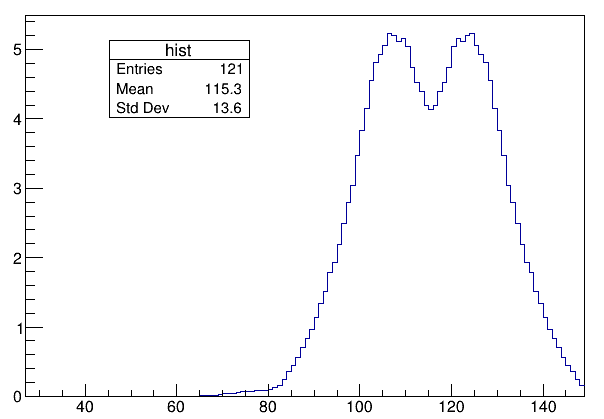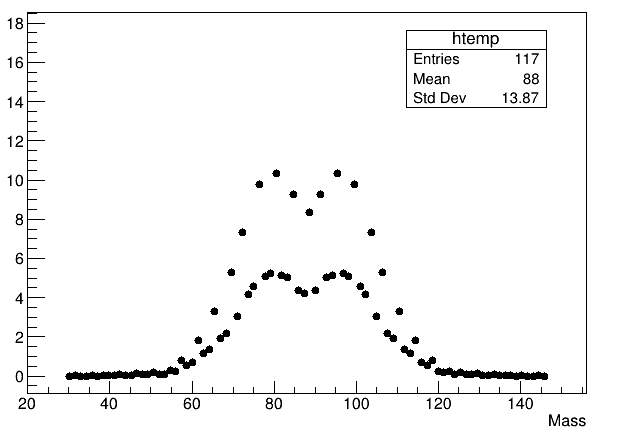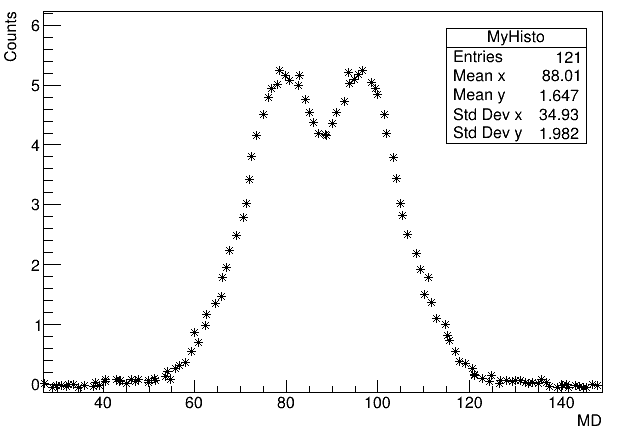# Unable to display the histogram entries and standard deviation

Hi,

When I run the following Macro, I am able to plot the data, unfortunately, I am not able to display the histogram entries and widths. Could someone please help me how to do this?

{
TTree *MyTree = new TTree(“MyTree”, “MyTree”);
MyTree->SetEstimate(-1);
MyTree->SetMarkerStyle(kFullStar);
MyTree->Draw(“Yield:Mass”);

}

The following is the output I get :I am also attaching the text file .
yield176_40.txt (6.1 KB)

Please read tips for efficient and successful posting and posting code

ROOT Version: Not Provided
Platform: Not Provided
Compiler: Not Provided

You need to create your own 2D histogram (so check the bins you need in x and y) and fill it when you do tree->Draw.

Your data file seems to be good for a “graph” only:

``````{
TGraph *g = new TGraph("yield176_40.txt");
g->SetTitle("Yield versus Mass;Mass [amu];Yield [%]");
g->SetMarkerStyle(kFullDotMedium);
g->Draw("ALP");
}
``````

I tried this, but the details of the histogram ie., the entries and the standard deviation are 0.

I had also tried using TGraph, attaching the macro below :
{
TGraph *graph = new TGraph(“yield176_30.dat”);

auto binEdges = graph->GetX();
auto values = graph->GetY();

auto hist = new TH1F(“hist”, “”, graph->GetN() - 1, binEdges);
//Fill the histogram
for (int i = 0; i < values->size(); i++) {
hist->SetBinContent(binEdges[i], values[i]);
}
hist->Draw("");
}

But it shows the following error when I try to compile it :

Your data file does not provide “errors” of “data points”.
Your “y-axis” seems to contain “percent values”, so the “number of entries” is not known either (for this data file and the “graph”, you could try `std::cout << g->Integral() << std::endl;`, thought I’m not sure if it really makes sense).

Yes, unfortunately, I don’t have the errors. I am really interested in the widths of the histogram. I have tried different methods, but somehow it’s not working correctly I would say :

METHOD I
{

TTree *tree = new TTree(“tree”,“tree”);
float bin, value;

//Get the bin edges and values out of the tree
std::vector binEdges, values;
for (int i = 0; i < tree->GetEntries(); i++) {
tree->GetEntry(i);
binEdges.push_back(bin);
values.push_back(value);
}

auto hist = new TH1F(“hist”, “”, binEdges.size() - 1, binEdges.data());
//Fill the histogram
for (int i = 0; i < values.size(); i++) {
hist->SetBinContent(binEdges.at(i), values.at(i));
hist->GetXaxis()->SetRangeUser(0,200);

}
hist->Draw("");
}

Here somehow the plot is truncated :METHOD II

TTree *MyTree = new TTree(“MyTree”, “MyTree”);
MyTree->SetEstimate(-1);
MyTree->Draw(“Mass”, “Yield”, “C”);
//gStyle->SetOptStat(111111);
}

And I get the output which is very weird.METHOD III

Also tried plotting 2D histogram

{
TTree *MyTree = new TTree(“MyTree”, “MyTree”);
MyTree->SetEstimate(MyTree->GetEntries()); // not strictly necessary
TH2F *MyHisto = new TH2F(“MyHisto”, “;MD;Counts”, 100, MyTree->GetMinimum(“MD”) - 1, MyTree->GetMaximum(“MD”) + 1, 100, MyTree->GetMinimum(“Counts”) - 1, MyTree->GetMaximum(“Counts”) + 1);

MyTree->Project(“MyHisto”, “Counts:MD”);
MyHisto->Draw("*");
}

and I get the following output where the widths of the mass distribution shown is not correctWhat I want to do is plot a 1D histogram and get the standard deviation of my mass distribution. But different methods give different widths. So what is the correct and reliable way to do this

For the “graph”, you can (though again, I’m not sure if it really makes sense):

``````std::cout << "mean x = " << g->GetMean(1) << std::endl;
std::cout << "mean y = " << g->GetMean(2) << std::endl;
std::cout << "rms x = " << g->GetRMS(1) << std::endl;
std::cout << "rms y = " << g->GetRMS(2) << std::endl;
``````

The “correct way” is to fit the data points, from the TGraph points in this case. Since you seem to have two peaks, there might be an issue with the data, but if this is right, you may probably need to fit 2 Gaussians (plus maybe background)…

``````{
TGraph *g = new TGraph("yield176_40.txt");
g->SetTitle("Yield versus Mass;Mass [amu];Yield [%]");
g->SetMarkerStyle(kFullDotMedium);
g->Sort(); // just a precaution
// g->Print();
TH1F *h = new TH1F("h", "Yield versus Mass;Mass [amu];Yield [%]", g->GetN(),
g->GetX() - 0.5, g->GetX()[(g->GetN() - 1)] + 0.5);
// for (int i = 0; i < g->GetN(); i++) h->Fill(g->GetX()[i], g->GetY()[i]);
h->FillN(g->GetN(), g->GetX(), g->GetY());
// h->Sumw2(kFALSE); // restore "sqrt(contents)" errors
// h->ResetStats(); // reset the statistics including the number of entries
// h->Print("all");
gStyle->SetOptStat("iRMe");
TCanvas *c = new TCanvas("c", "c");
c->Divide(1, 2);
c->cd(1);
g->Draw("ALP");
c->cd(2);
h->Draw("HIST");
c->cd(0);
}
``````

This is amazing! It works fine now.

Thanks a lot Wile @Wile_E_Coyote.

@Wile_E_Coyote, I had one more doubt here, if I want to see the effect of my experimental mass resolution of 3.5 amu on the widths of my theoretical mass distribution (which is the one we plot from the text file), how do I do that?

Here, in the “yield176_40.txt”, the first column is the theoretical mass (in amu) and the second column is its weight.